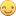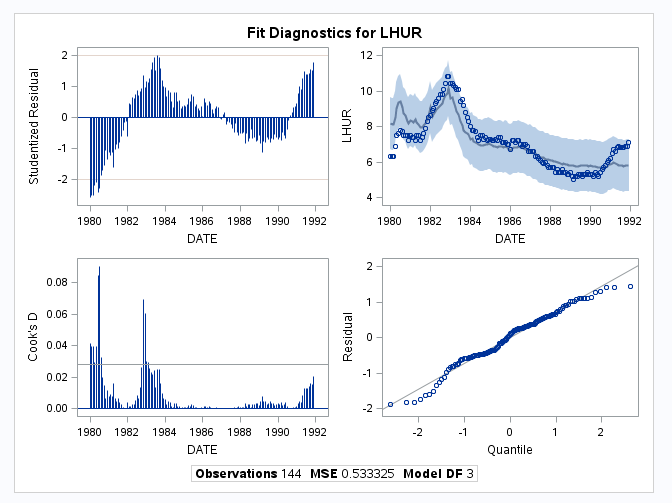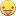## PROC MODEL: How can I get the data for the Cook's D graph in an output dataset

First a small disclaimer: I am asking this question as a statistical noob on behalf of a custormer of ours.We are running SAS 9.4 M3 (SAS/ETS 14.1)

The customer runs various fit analysis using PROC MODEL and one of the graphs that is being generated is the Cook's D graph

The question of the customer is: can I get the data that is being used to generate the Cook's D graph in an output dataset and link this back to my source data so I can filter outliers?

By using the ODS TRACE statement I have been able to derive - I think - the base data that is being used.

The code I have used (hopefully as a representative example) is:

``````ods trace on;
proc model data=sashelp.citimon;
lhur = 1/(a * ip + b) + c;
fit lhur;
id date;
run;
ods trace off;``````

Attached a sample of part the output that is generated, specifically Panel 1 that contains the Cook's D graph.

Comparing the output in the log against the output my assumption is that the base data for the graphs can be obtained by adding the following statement just before the PROC MODEL statement:

``ods output DiagnosticsPanel=work.panel;``

However, it seems as if the dataset contains all the data (the dataset contains 36 variables) that is needed to create the graphs in Panel 1 (and 2?) and I could find no way to relate the data back to the source data. I was hoping that adding the option PLOT(ONLY)=COOKSD would reduce the number of variables in the output dataset but it - as maybe could be expected- only reduced the number of graphs.

Hopefully someone can shed some light on this and help me help our customer.

TIA1 ACCEPTED SOLUTION

Accepted Solutions

## Re: PROC MODEL: How can I get the data for the Cook's D graph in an output dataset

The dataset includes two variables to help you match back to the original:

ID - count of obs from 1 to NObs

Actual - the observed value

_____RESIDUAL_RESIDUAL____MSE_WE - I think this is the Cook's D variable.

@Resa wrote:

First a small disclaimer: I am asking this question as a statistical noob on behalf of a custormer of ours.We are running SAS 9.4 M3 (SAS/ETS 14.1)

The customer runs various fit analysis using PROC MODEL and one of the graphs that is being generated is the Cook's D graph

The question of the customer is: can I get the data that is being used to generate the Cook's D graph in an output dataset and link this back to my source data so I can filter outliers?

By using the ODS TRACE statement I have been able to derive - I think - the base data that is being used.

The code I have used (hopefully as a representative example) is:

``````ods trace on;
proc model data=sashelp.citimon;
lhur = 1/(a * ip + b) + c;
fit lhur;
id date;
run;
ods trace off;``````

Attached a sample of part the output that is generated, specifically Panel 1 that contains the Cook's D graph.

Comparing the output in the log against the output my assumption is that the base data for the graphs can be obtained by adding the following statement just before the PROC MODEL statement:

``ods output DiagnosticsPanel=work.panel;``

However, it seems as if the dataset contains all the data (the dataset contains 36 variables) that is needed to create the graphs in Panel 1 (and 2?) and I could find no way to relate the data back to the source data. I was hoping that adding the option PLOT(ONLY)=COOKSD would reduce the number of variables in the output dataset but it - as maybe could be expected- only reduced the number of graphs.

Hopefully someone can shed some light on this and help me help our customer.

TIA

2 REPLIES 2

## Re: PROC MODEL: How can I get the data for the Cook's D graph in an output dataset

The dataset includes two variables to help you match back to the original:

ID - count of obs from 1 to NObs

Actual - the observed value

_____RESIDUAL_RESIDUAL____MSE_WE - I think this is the Cook's D variable.

@Resa wrote:

First a small disclaimer: I am asking this question as a statistical noob on behalf of a custormer of ours.We are running SAS 9.4 M3 (SAS/ETS 14.1)

The customer runs various fit analysis using PROC MODEL and one of the graphs that is being generated is the Cook's D graph

The question of the customer is: can I get the data that is being used to generate the Cook's D graph in an output dataset and link this back to my source data so I can filter outliers?

By using the ODS TRACE statement I have been able to derive - I think - the base data that is being used.

The code I have used (hopefully as a representative example) is:

``````ods trace on;
proc model data=sashelp.citimon;
lhur = 1/(a * ip + b) + c;
fit lhur;
id date;
run;
ods trace off;``````

Attached a sample of part the output that is generated, specifically Panel 1 that contains the Cook's D graph.

Comparing the output in the log against the output my assumption is that the base data for the graphs can be obtained by adding the following statement just before the PROC MODEL statement:

``ods output DiagnosticsPanel=work.panel;``

However, it seems as if the dataset contains all the data (the dataset contains 36 variables) that is needed to create the graphs in Panel 1 (and 2?) and I could find no way to relate the data back to the source data. I was hoping that adding the option PLOT(ONLY)=COOKSD would reduce the number of variables in the output dataset but it - as maybe could be expected- only reduced the number of graphs.

Hopefully someone can shed some light on this and help me help our customer.

TIA

## Re: PROC MODEL: How can I get the data for the Cook's D graph in an output dataset

Hi @Reeza,

Based on your information (created a needle plot using the variable you indicated) it seems you have this correct.

I will confirm with the customer tomorrow and if your information is correct will mark your reply as a solution.

It would have been nice if they would have given the column a somewhat more meaningfull name thoughWill keep you posted

--Resa

Discussion stats
• 2 replies
• 936 views
• 1 like
• 2 in conversation